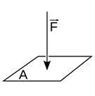# The Concept of Pressure

## What is Pressure?

Have you ever wondered why our knives need to be so sharp or why the nails we use the end with a sharp point? The answers to all these questions lie in the concept of pressure. It is the ratio of the force applied to the surface area over which the force is applied. We can define pressure as:

The force applied perpendicular to the surface of an object per unit area over which that force is distributed.### Formula:

When a force of ‘F’ Newton is applied perpendicularly to a surface area ‘A’, then the pressure exerted on the surface by the force is equal to the ratio of F to A. The formula for pressure (P) is:

P = F / A### Units of Pressure

There are various units to describe Pressure some of which we will discuss further in this article.

The SI unit of pressure is the pascal (Pa).

A pascal can be defined as a force of one newton applied over a surface area of a one-meter square.

You may also want to check out these topics given below!

## Factors Affecting Pressure

Since the pressure is dependent on the area over which the force is acting, the pressure can be increased and decreased without any change in the force. The force applied to be constant if the surface becomes smaller the pressure increases and vice versa.

For example, a brick sitting on a surface exerts a force equal to its weight on the object it is resting on. Now we know that a rectangular brick has a wide surface and a thin surface on the sides. By changing the orientation of the brick resting on a surface, we are effectively changing the pressure acting on the surface by the same brick. See the image below for more information.In other words, if the surface becomes smaller, the pressure becomes larger. It is for this reason that our knives and nails are so sharp. A knife distributes the force over its entire cutting edge. Sharper the edge, higher the pressure, and consequently the cutting with a sharp knife is easy. In a blunt knife, the force is distributed over its blunt surface with a larger surface area. Therefore, we need to put more force in order to cut. Therefore, a knife is best when at its sharpest.

For the same reason—that is, that reduction of surface area increases net pressure—an expertly delivered karate chop is much more damaging and deadly than an open-handed slap. When you slap someone, the force you apply in slapping the surface is distributed all over the palm of your hand. In contrast, a karate chop concentrates all the force on the sides of your hand which have significantly lesser surface area than your palms. This leads to a greater application of pressure on the surface thereby rendering a karate chop is deadlier than a slap.

Sometimes though, a greater surface area is also preferred. A typical drawing pin comes with one flat round end with which you push the other sharp end into the drawing board. Can you imagine how hard it would be to push a drawing pin into a board if both its sides were sharp? Since one end is flat, you can apply the necessary force easily. Similar tactics are used in skiing and surfing. By using surfboards and skis, we increase the area over which our weight acts thereby allowing us to float or glide over the surface of water or ice.

## Frequently Asked Questions – FAQs

Q1

### Define pressure.

The force applied perpendicular to the surface of an object per unit area over which that force is distributed is known as pressure.
Q2

### Define force.

The push or pull on an object with mass that causes it to change its velocity is known as force.
Q3

### Define pascal.

A pascal can be defined as a force of one newton applied over a surface area of a one-meter square.
Q4

### Why do fluids exerts pressure?

The fluid exerts pressure in all directions because the particles in the fluid can move in all directions. The movement of these molecules results in continuous collisions. The collision causes pressure which is exerted in all directions.
Q5

### How does temperature affect gas pressure?

We know that the temperature of the gas is proportional to the average kinetic energy of the molecules. As the kinetic energy increases, the collision between the molecules increases resulting in increased pressure in the gas.